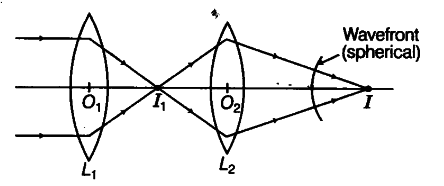# Consider a point at the focal point of convergent lens

Consider a point at the focal point of convergent lens. Another convergent lens of short focal length is placed on the other side. What is the nature of the wavefronts emerging from the final image?

Consider the ray diagram shown belowThe point image\$I_{1 }\$ due to L_{ 1 } is at the focal point. Now, due to the converging lens\$L_{ 2}\$, let the final image formed be I which is a point image, hence the wavefront for this image will be of spherical symmetry.